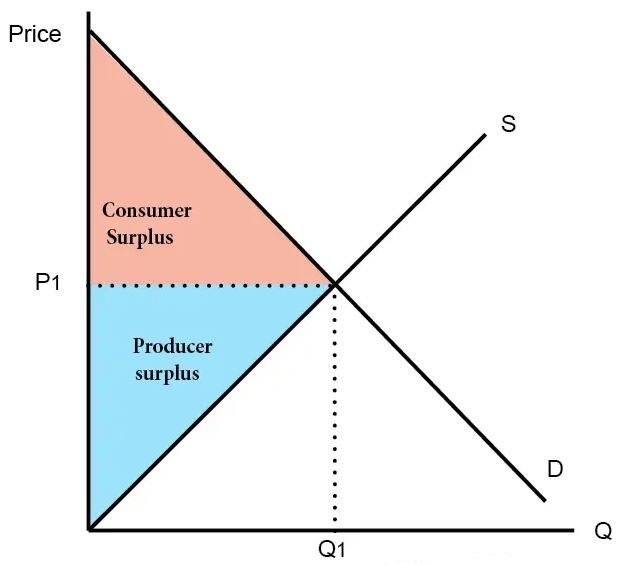# Consumer Surplus Calculator

Created by Tibor Pal, PhD candidate
Reviewed by Dominik Czernia, PhD candidate and Jack Bowater
Based on research by
Krugman, P.; Wells R. Economics, Fifth Edition (2017)
Last updated: Mar 11, 2022

The consumer surplus calculator is a handy tool that helps you to compute the difference between what consumers are willing to pay for a good or service, versus its market price. If you read on, you can learn what consumer surplus is, based on the consumer surplus definition. We will also show you how to find consumer surplus using the consumer surplus formula.

## What is consumer surplus? - the consumer surplus definition and graph

In economics, consumer surplus is the difference between the price that consumers actually pay, and the maximum price that they are willing to pay. If you have ever attended an economics lecture, you have probably seen curves representing supply (S) and demand (D) as a function of price (P) versus quantity (Q).

If not, you can quickly grasp the concept in the consumer surplus graph below. The demand curve (D) is downward, as a lower price implies a higher demand for a given article. The supply curve (S), in contrast, is upward, since manufacturers produce more if they can sell their product at a higher price. The consumer surplus is the area between the equilibrium price (the level of price where the two curves cross each other) and the demand curve.## The consumer surplus formula

Now that you know what a consumer surplus is, let's find out how to calculate consumer surplus. According to the consumer surplus definition we talk about above, you've probably already figured out what is the consumer surplus formula. Indeed, it is the following simple equation:

consumer surplus = maximum price willing to pay - actual market price.

If you would like to estimate the consumer surplus for a whole economy, you need to use a slightly extended version of the formula, which you can reach in the advanced mode of this consumer surplus calculator.

Extended Consumer Surplus = 0.5 * Qd - Pmax - Pd,

where:

• Qd is the quantity demanded at equilibrium, where demand and supply are equal;
• Pmax is the maximum price the buyer is willing to pay; and
• Pd is the price at equilibrium, where demand and supply are equal.

## How to calculate consumer surplus? - an example

Let's look at an example to make sure that you've understood the concept behind the consumer surplus.

You have decided to buy a football, and you are willing to pay $100 for it. You check on the Internet for their prices there, and you find a shop where you can buy it for$80.

This means that you can save $20 on the purchase compared to what you were willing to spend. The$20 is your consumer surplus, which you can now save, invest or spend on other goods and services.

## How to use the consumer surplus calculator?

You get the value of the consumer surplus immediately after setting the actual price, and the maximum price that the buyer willing to pay (willing price). Alternatively, you can set the consumer surplus, and give one of the other two parameters to receive the missing one.

In the advanced mode, you can set the equilibrium price, and the equilibrium quantity (the values where the supply and demand equal), and you can read the value for the extended consumer surplus.

Tibor Pal, PhD candidate
Actual Price
$Willing Price$
Consumer Surplus
\$
People also viewed…

### BMR - Harris-Benedict equation

Harris-Benedict calculator uses one of the three most popular BMR formulas. Knowing your BMR (basal metabolic weight) may help you make important decisions about your diet and lifestyle.

### Debt consolidation

Use the debt consolidation calculator to compare your current debts with a given consolidating loan.

### Rate of return

The rate of return calculator is a tool that helps you calculate rate of return - a measurement of a profitability of an investment.

### Sunbathing

Do you always remember to put on sunscreen before going outside? Are you sure that you use enough? The Sunbathing Calculator ☀ will tell you when's the time to go back under an umbrella not to suffer from a sunburn!